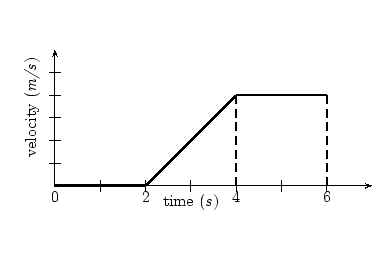# 8.1: Unit 8 Practice and Assessment

$$\newcommand{\vecs}{\overset { \rightharpoonup} {\mathbf{#1}} }$$ $$\newcommand{\vecd}{\overset{-\!-\!\rightharpoonup}{\vphantom{a}\smash {#1}}}$$$$\newcommand{\id}{\mathrm{id}}$$ $$\newcommand{\Span}{\mathrm{span}}$$ $$\newcommand{\kernel}{\mathrm{null}\,}$$ $$\newcommand{\range}{\mathrm{range}\,}$$ $$\newcommand{\RealPart}{\mathrm{Re}}$$ $$\newcommand{\ImaginaryPart}{\mathrm{Im}}$$ $$\newcommand{\Argument}{\mathrm{Arg}}$$ $$\newcommand{\norm}{\| #1 \|}$$ $$\newcommand{\inner}{\langle #1, #2 \rangle}$$ $$\newcommand{\Span}{\mathrm{span}}$$ $$\newcommand{\id}{\mathrm{id}}$$ $$\newcommand{\Span}{\mathrm{span}}$$ $$\newcommand{\kernel}{\mathrm{null}\,}$$ $$\newcommand{\range}{\mathrm{range}\,}$$ $$\newcommand{\RealPart}{\mathrm{Re}}$$ $$\newcommand{\ImaginaryPart}{\mathrm{Im}}$$ $$\newcommand{\Argument}{\mathrm{Arg}}$$ $$\newcommand{\norm}{\| #1 \|}$$ $$\newcommand{\inner}{\langle #1, #2 \rangle}$$ $$\newcommand{\Span}{\mathrm{span}}$$$$\newcommand{\AA}{\unicode[.8,0]{x212B}}$$

## Outcome 1

1. Explain the difference between distance and displacement.
2. Explain how velocity relates to position.
3. Explain how acceleration relates to velocity.

## Outcome 2

1. Calculate the drag force on a swimmer moving through water at 0.75 m/s. The drag coefficient for a human in the prone position is roughly 0.25. Look up for the density of water in standard units and cite your source. Estimate the cross-sectional area of a human for this situation by using the width and depth of your own body or average human body measurements (cite your source).
1. The swimmer above is moving at a constant speed. What is the average forward-directed force applied to the swimmer by the water due to their swimming motion?

## Outcomes 3, 4

1. A toddler runs away from a parent at 0.3 m/s for 3 s, stops for 2 s to see if they are being chased.

a) Draw a velocity vs. time graph for the toddler’s motion

b) Draw an acceleration vs. time graph for the toddler’s motion

c) Draw a position vs. time graph for the toddler’s motion (you will need to calculate the displacements that occur during each interval in order to draw this graph).

1. Upon realizing they might be chased after the 2 s stop, the toddler from the previous exercise begins slowly walking away and increasing speed into a run, reaching a speed of 0.4 m/s only 3 s later.

a) Complete the acceleration vs. time graph for the toddler’s motion, now including this new motion. You may draw a new graph or add to your previous graph in a different color. (You will need to calculate the acceleration during this last part of the toddler’s motion in order to complete this graph).

b) Complete the velocity vs. time graph for the toddler’s motion. You may draw a new graph or add to your previous graph in a different color. (You will need to use the acceleration you found above to calculate a change in velocity to complete this graph).

c) Complete the position vs. time graph for the toddler’s motion. You may draw a new graph or add to your previous graph in a different color. (You will need to use the acceleration you found above to calculate displacements to complete this graph).

1. Describe the motion depicted by the following velocity vs. time graph. The vertical axis tick marks indicate 1 m/s intervals, starting from zero m/s at the horizontal axis.Velocity vs. time graph. The vertical axis marks indicate 1 m/s intervals. Image Credit: Uploaded by Riaan at English Wikibooks.

6) Draw the acceleration vs. time graph associated with the velocity vs time graph above.

7) Draw the position vs. time graph associated with the previous velocity and acceleration vs. time graphs.

## Outcome 4

8) A person with mass of 65 kg is out walking two dogs and stops to talk with a friend. Suddenly the dogs pull in opposite directions. Dog 1 pulls with a force of 500 N to the right. Dog 2 pulls with 300 N to the left.

a) Draw a free body diagram of the dog walker.

b) What is the net force on the dog walker?

c) What is the acceleration of the dog walker, including direction.

d) What distance will the dog walker have moved in 3 s?

e) What will the velocity of the dog walker be after 3 s?